# Allegation or Mixture

## Alligation

 Question 1
The cost of Type 1 rice is Rs. 15 per kg and Type 2 rice is Rs. 20 per kg. If both Type 1 and Type 2 are mixed in the ratio of 2 : 3, then the price per kg of the mixed variety of rice is:
 A Rs. 18.50 B Rs. 19.50 C Rs. 19 D Rs. 18
Question 1 Explanation:Question 2
A can contains a mixture of two liquids A and B is the ratio 7 : 5. When 9 litres of mixture are drawn off and the can is filled with B, the ratio of A and B becomes 7 : 9. How many litres of liquid A was contained by the can initially?
 A 10 B 20 C 21 D 25
Question 2 Explanation:
\begin{align} & {\text{Suppose}}\,{\text{the}}\,{\text{can}}\,{\text{initially}}\,{\text{contains}}\, \cr & 7x\,{\text{and}}\,5x\,{\text{of}}\,{\text{mixtures}}\,{\text{A}}\,{\text{and}}\,{\text{B}}\,{\text{respectively}}. \cr & {\text{Quantity}}\,{\text{of}}\,{\text{A}}\,{\text{in}}\,{\text{mixture}}\,{\text{left}} \cr & = \left( {7x - \frac{7}{{12}} \times 9} \right)\,{\text{litres}} \cr & = \left( {7x - \frac{{21}}{4}} \right)\,{\text{litres}} \cr & {\text{Quantity}}\,{\text{of}}\,{\text{B}}\,{\text{in}}\,{\text{mixture}}\,{\text{left}} \cr & = \left( {5x - \frac{5}{{12}} \times 9} \right)\,{\text{litres}} \cr & = \left( {5x - \frac{{15}}{4}} \right)\,{\text{litres}} \cr & \therefore \frac{{\left( {7x - \frac{{21}}{4}} \right)}}{{\left( {5x - \frac{{15}}{4}} \right) + 9}} = \frac{7}{9} \cr & \Rightarrow \frac{{28x - 21}}{{20x + 21}} = \frac{7}{9} \cr & \Rightarrow 252x - 189 = 140x + 147 \cr & \Rightarrow 112x = 336 \cr & \Rightarrow x = 3 \cr\end{align}
 Question 3
Tea worth Rs. 126 per kg and Rs. 135 per kg are mixed with a third variety in the ratio 1 : 1 : 2. If the mixture is worth Rs. 153 per kg, the price of the third variety per kg will be:
 A Rs. 175.50 B Rs. 170 C Rs. 180 D Rs. 169.50
Question 3 Explanation:
Since first and second varieties are mixed in equal proportions.

So, their average price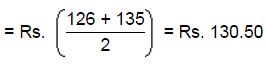So, the mixture is formed by mixing two varieties, one at Rs. 130.50 per kg and the other at say, Rs. x per kg in the ratio 2 : 2, i.e., 1 : 1. We have to find x.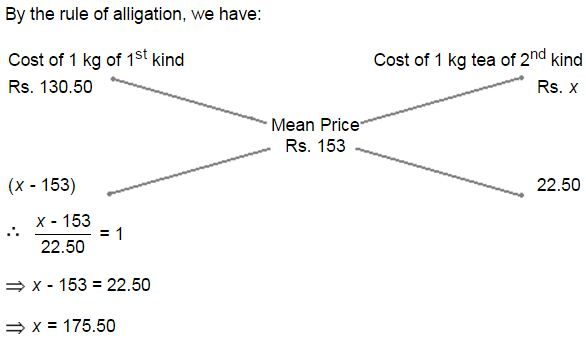Question 4
Weights of two friends Ram and Shyam are in the ratio 4:5. If Ram's weight is increased by 10% and total weight of Ram and Shyam become 82.8 kg, with an increases of 15%. By what percent did the weight of Shyam has to be increased?
 A 10% B 16% C 19% D 21%
Question 4 Explanation: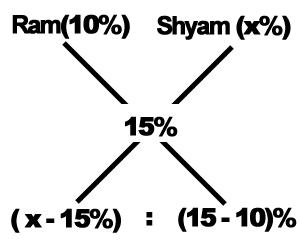Now, given ratio of Ram and Shayam's weight = 4:5

Hence, (x-15)/(15-10) = 4/5

Or, x = 19%

 Question 5
In a zoo, there are Rabbits and Pigeons. If heads are counted, there are 200 and if legs are counted, there are 580. How many pigeons are there?
 A 110 B 100 C 90 D 120
Question 5 Explanation:

Legs count = 580.

Average Legs count for per head = 580/200 = 29/10.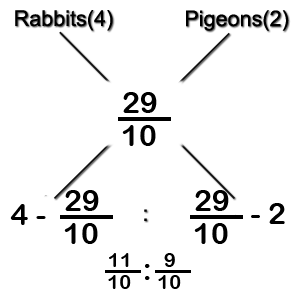Rabbits : Pigeons = (9/10) : (11/10)= 9 : 11.

Number of Pigeons = (200 *11)/20 = 110.

 Question 6
The ratio, in which tea costing Rs. 192 per kg is to be mixed with tea costing Rs. 150 per kg so that the mixed tea when sold for Rs. 194.40 per kg, gives a profit of 20%.
 A 3:5 B 2:5 C 5:3 D 5:2
Question 6 Explanation:
CP of first tea = Rs. 192 per kg.

CP of Second tea = Rs. 150 per kg.

Mixture is to be sold in Rs. 194.40 per kg, which has included 20% profit. So,

SP of Mixture = Rs. 194.40 per kg.

Let the CP of Mixture be Rs. X per kg. Therefore,

X + 20% of X = SP

6X /5 = 194.40

6X = 194.40 *5

X = Rs. 162 per kg.

Let N kg of first tea and M kg of second tea to be added.

Now, Using Alligation,

We get,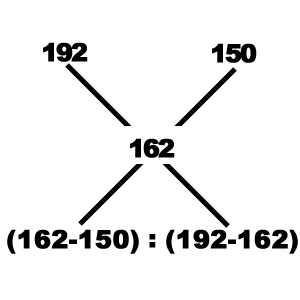N/M = 12/30

N : M = 2 : 5.

 Question 7
In a zoo, there are deers and ducks. If the heads are counted, there are 180, while the legs are 448. What will be the number of deers in the zoo?
 A 68 B 22 C 44 D 136
Question 7 Explanation:
Average legs per head = 448/180.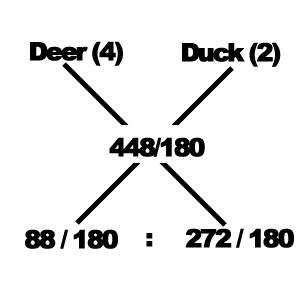Deers: Ducks = 88: 272

Hence,the number of Deers = (180*88)/360 = 44.

 Question 8
3 L water is taken out from vessel full of water and substituted by pure milk. This process is repeated two more times. Finally, the ratio of milk and water in the solution becomes 1728:27. Find the volume of the original solution.
 A 3 L B 5 L C 9 L D 4 L
Question 8 Explanation:

We use formula for it where

n = no. of time process of out going repeated.

y = Out going milk in process.

x = Volume of original solution.

F = Final milk left in mixture.

Final quantity of water,

= x[1-(y/x)]n

= x[1-(3/x)]3

F/x = x[1-(3/x)]3

27/1728 = x[1-(3/x)]3

Or, x = 4 L.

 Question 9
Find the ratio in which rice at Rs. 7.20 a kg be mixed with rice at Rs. 5.70 a kg to produce a mixture worth Rs. 6.30 a kg.
 A 3 : 4 B 2 : 3 C 4 : 5 D 1 : 3
Question 9 Explanation: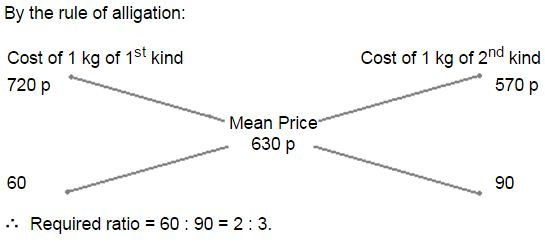Question 10
An amount of Rs. 680 was invested at 6% rate of interest and another sum of money was invested at 10% interest. If the average interest on the total at the end of the year was 7.5%, how much was invested at 10%?
 A Rs. 408 B Rs. 267.5 C Rs. 412.6 D None of these
Question 10 Explanation: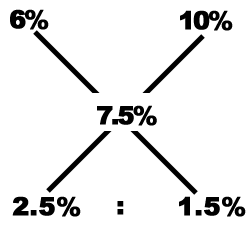Now, 680/x = 2.5/1.5

Or, x = (680*3)/5

Hence, x = Rs. 408.

There are 10 questions to complete.